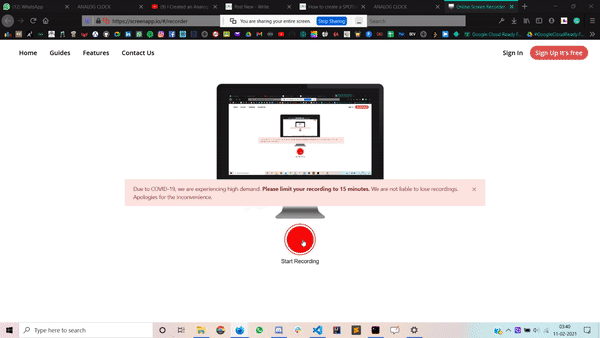Related Articles

# Responsive analog clock using HTML, CSS and Vanilla JavaScript

• Last Updated : 04 Mar, 2021

In this article, we are going to create an Analog Clock. This is mainly based on HTML, CSS & Vanilla JavaScript.

Approach:

1. Create an HTML file in which we are going to add the main div, further on we are adding 4 div tags for an hour, minute, second hands & for the pin.
2. Create a CSS file for styling our web-page and for assigning different lengths to the different hands.
3. Create a JavaScript file for creating a brief logic for rotation of different clock-hands.

Logic for rotation of clock hands:

1. Hour Hand

```For Achieving 12hrs,
hour hand moves 360deg.

i.e.  12hrs   ⇢   360degs

so,    1hr    ⇢   30degs

and, 60mins   ⇢   30degs

so,    1min   ⇢   0.5degs

Total Rotation of hour hand:
(30deg * hrs) + (0.5deg * mins)```

2. Minute Hand

```For Achieving 60mins,
hour hand moves 360deg.

i.e.  60mins   ⇢   360degs

so,    1min    ⇢   6degs

Total Rotation of minute hand:
6deg * mins```

3. Second Hand

```For Achieving 60secs,
hour hand moves 360deg.

i.e.  60secs   ⇢   360degs

so,    1sec    ⇢   6degs

Total Rotation of minute hand:
6deg * secs```

HTML Code:

## HTML

 ```<``html` `lang``=``"en"``>``   ` `<``head``>``    ``<``title``>Analog Clock``    ``<``link` `rel``=``"stylesheet"` `href``=``"style.css"``>````   ` `<``body``>``    ``<``div` `class``=``"clock"``>``        ``<``div` `class``=``"hr"``>``        ``<``div` `class``=``"min"``>``        ``<``div` `class``=``"sec"``>``        ``<``div` `class``=``"pin"``>``    ```` ` `    ``<``script` `src``=``"index.js"``>````   ` ``

Code Explanation:

• First, create an HTML file (index.html).
• Now after the creation of our HTML file, we are going to give a title to our webpage using <title> tag. It should be placed inside the <head> section.
• Then we link the CSS file that provides all the styles to our HTML. This is also placed in between the <head> tag.
• Coming to the body section of our HTML code.
• Firstly, create a main div as a clock.
• In that div add 4divs for an hour, minute, second hands & for the pin.
• At the end of our body add <script> tag which links the JS file with our HTML file.

CSS Code:

 `/* Restoring browser effects */``* {``    ``margin``: ``0``;``    ``padding``: ``0``;``    ``box-sizing: border-box;``    ``;``}`` ` `/* All of the same styling to the body */``body {``    ``height``: ``100``vh;``    ``display``: flex;``    ``justify-``content``: ``center``;``    ``align-items: ``center``;``    ``background-color``: ``#000``;``    ``background-image``: linear-gradient(``            ``70``deg, ``black``, ``white``);``}`` ` `/* Sizing, positioning of main ``    ``dial of the clock */``.clock {``    ``width``: ``40``vw;``    ``height``: ``40``vw;``    ``background-image``: linear-gradient(``                ``70``deg, ``black``, ``white``);``    ``background-``size``: cover;``    ``box-shadow: ``0` `3em` `5.8em``;``    ``border-radius: ``50%``;``    ``position``: ``relative``;``}`` ` `.hr,``.min,``.sec {``    ``width``: ``1%``;``    ``position``: ``absolute``;``    ``top``: ``50%``;``    ``left``: ``50%``;``    ``transform: translate(``-50%``, ``-100%``);``    ``transform-origin: ``bottom``;``    ``z-index``: ``2``;``    ``border-radius: ``2em``;``}`` ` `.pin {``    ``position``: ``absolute``;``    ``top``: ``0``;``    ``left``: ``0``;``    ``right``: ``0``;``    ``bottom``: ``0``;``    ``width``: ``1em``;``    ``height``: ``1em``;``    ``background``: ``rgb``(``38``, ``0``, ``255``);``    ``border``: ``2px` `solid` `#ffffff``;``    ``border-radius: ``10em``;``    ``margin``: ``auto``;``    ``z-index``: ``10``;``}`` ` `/* Different length of different hands of clock */``.hr {``    ``height``: ``25%``;``    ``background-color``: ``#ff0000``;``}`` ` `.min {``    ``height``: ``30%``;``    ``background-color``: ``#ff9900``;``}`` ` `.sec {``    ``height``: ``40%``;``    ``background-color``: ``#99ff00``;``    ``transform-origin: ``50%` `85%``;``}`

Code Explanation: CSS is used to give different types of animations and effects to our HTML page so that it looks interactive to all users. In CSS, we have to include the following points:

1. Restore all the browser effects.
2. Use classes and id’s to give effects to HTML elements.

JS Code:

## Javascript

 `// Selecting all of the css classes on which``// we want to apply functionalities``const hr = document.querySelector(``'.hr'``)``const min = document.querySelector(``'.min'``)``const sec = document.querySelector(``'.sec'``)`` ` `// Setting up the period of working``setInterval(() => {`` ` `    ``// Extracting the current time ``    ``// from DATE() function``    ``let day = ``new` `Date()``    ``let hour = day.getHours()``    ``let minutes = day.getMinutes()``    ``let seconds = day.getSeconds()`` ` `    ``// Formula that is explained above for ``    ``// the rotation of different hands``    ``let hrrotation = (30 * hour) + (0.5 * minutes);``    ``let minrotation = 6 * minutes;``    ``let secrotation = 6 * seconds;`` ` `    ``hr.style.transform =``        ```translate(-50%,-100%) rotate(\${hrrotation}deg)```    ``min.style.transform =``        ```translate(-50%,-100%) rotate(\${minrotation}deg)```    ``sec.style.transform =``        ```translate(-50%,-85%) rotate(\${secrotation}deg)```});`

Code Explanation:

• The setInterval() function is used for the execution of function for a specific period of time. For more details click here.
• The Date() function is used for returning today date, current time(hours, minutes, seconds).

Complete Code:

## HTML

 ```<``html` `lang``=``"en"``>`` ` `<``head``>``    ``<``style``>`` ` `        ``/* Restoring browser effects */        ``        ``* {``            ``margin: 0;``            ``padding: 0;``            ``box-sizing: border-box;``        ``}`` ` `        ``/* All of the same styling to the body */        ``        ``body {``            ``height: 100vh;``            ``display: flex;``            ``justify-content: center;``            ``align-items: center;``            ``background-color: #000;``            ``background-image: linear-gradient(``                ``70deg, black, white);``        ``}`` ` `        ``/* Sizing, positioning of main dial of the clock */        ``        ``.clock {``            ``width: 40vw;``            ``height: 40vw;``            ``background-image: linear-gradient(``                ``70deg, black, white);``            ``background-size: cover;``            ``box-shadow: 0 3em 5.8em;``            ``border-radius: 50%;``            ``position: relative;``        ``}``         ` `        ``.hr,``        ``.min,``        ``.sec {``            ``width: 1%;``            ``position: absolute;``            ``top: 50%;``            ``left: 50%;``            ``transform: translate(-50%, -100%);``            ``transform-origin: bottom;``            ``z-index: 2;``            ``border-radius: 2em;``        ``}``         ` `        ``.pin {``            ``position: absolute;``            ``top: 0;``            ``left: 0;``            ``right: 0;``            ``bottom: 0;``            ``width: 1em;``            ``height: 1em;``            ``background: rgb(38, 0, 255);``            ``border: 2px solid #ffffff;``            ``border-radius: 10em;``            ``margin: auto;``            ``z-index: 10;``        ``}`` ` `        ``/* Different length of different hands of clock */        ``        ``.hr {``            ``height: 25%;``            ``background-color: #ff0000;``        ``}``         ` `        ``.min {``            ``height: 30%;``            ``background-color: #ff9900;``        ``}``         ` `        ``.sec {``            ``height: 40%;``            ``background-color: #99ff00;``            ``transform-origin: 50% 85%;``        ``}``    `````` ` `<``body``>``    ``<``div` `class``=``"clock"``>``        ``<``div` `class``=``"hr"``>``        ``<``div` `class``=``"min"``>``        ``<``div` `class``=``"sec"``>``        ``<``div` `class``=``"pin"``>``    ```` ` `    ``<``script``>`` ` `        ``// Selecting all of the css classes ``        ``// on which we want to apply functionalities``        ``const hr = document.querySelector('.hr')``        ``const min = document.querySelector('.min')``        ``const sec = document.querySelector('.sec')`` ` `        ``// Setting up the period of working``        ``setInterval(() => {`` ` `            ``// Extracting the current time ``            ``// from DATE() function``            ``let day = new Date()``            ``let hour = day.getHours()``            ``let minutes = day.getMinutes()``            ``let seconds = day.getSeconds()`` ` `            ``// Formula that is explained above for ``            ``// the rotation of different hands``            ``let hrrotation = (30 * hour) + (0.5 * minutes);``            ``let minrotation = 6 * minutes;``            ``let secrotation = 6 * seconds;`` ` `            ``hr.style.transform =``                ```translate(-50%,-100%) rotate(\${hrrotation}deg)```            ``min.style.transform =``                ```translate(-50%,-100%) rotate(\${minrotation}deg)```            ``sec.style.transform =``                ```translate(-50%,-85%) rotate(\${secrotation}deg)```        ``});``    `````` ` ``

Output:Attention reader! Don’t stop learning now. Get hold of all the important HTML concepts with the Web Design for Beginners | HTML  course.

My Personal Notes arrow_drop_up